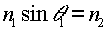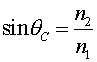The critical angle for total internal reflection

When light leaves a medium of higher index n1 and enters one of lower index n2, (e.g. light escaping glass and going into the air) the angle of refraction will be larger than the angle of incidence. Hence the outgoing ray will be bent towards the surface. As the angle of incidence is increased, the angle of refraction will eventually reach 90 degrees. Beyond that angle of incidence, Snell's law requires sinq2 to be greater than unity. When that occurs, there is no mathematical solution for the outgoing angle, and hence there is no refracted ray at all. Instead, all of the light (instead of just some of it) is reflected from the surface. To solve for this critical angle of incidence q1, consider Snell's law with sinq2 set equal to unity:This gives a formula for the critical angle qc,The phenomenon of total internal reflection is the basis for fiber optic cables, which are used to carry signals over very long distances with very little loss in intensity.

Refraction index      examples        Lecture index